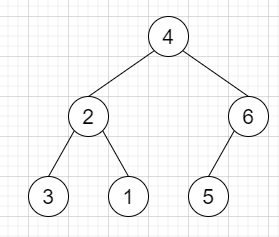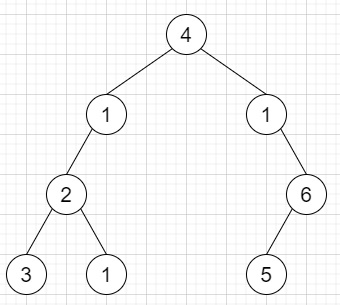# Add One Row to Tree in C++

Suppose we have a binary tree, we also have the value v and depth d, we have to add a row of nodes with value v at the given depth d. The root node is at depth 1. We have to follow this rule to perform this operation −

As we know the depth d, for each valid tree nodes N in depth d-1, we have to create two tree nodes with value v as N's left subtree root and right subtree root. And N's original left subtree will be the left subtree of the new left subtree root, its original right subtree will be the right subtree of the new right subtree root. When the depth d is 1 that means there is no depth d-1 at all, then create a tree node with value v as the new root of the whole original tree, and the original tree is the new root's left subtree.

So, if the input is likethen the output will beTo solve this, we will follow these steps −

• if d is same as 1, then −

• temp = new node with value v

• left of temp := root

• root := temp

• Otherwise

• Define one stack of pairs called st

• insert { root, 2 } into st

• lvl := 0

• Define one pair temp

• while (not st is empty), do −

• temp := top element of st

• delete element from st

• lvl := second element of temp

• node := first element of temp

• if lvl is same as d, then −

• temp1 = new node with value v

• temp2 = new node with value v

• left of temp1 := left of node

• right of temp2 := right of node

• left of node := temp1

• right of node := temp2

• Otherwise

• if left of node is valid, then −

• insert {left of node, lvl + 1} into st

• if right of node is valid, then −

• insert {right of node, lvl + 1} into st

• return root

## Example

Let us see the following implementation to get better understanding −

Live Demo

#include <bits/stdc++.h>
using namespace std;
class TreeNode{
public:
int val;
TreeNode *left, *right;
TreeNode(int data){
val = data;
left = NULL;
right = NULL;
}
};
void insert(TreeNode **root, int val){
queue<TreeNode*> q;
q.push(*root);
while(q.size()){
TreeNode *temp = q.front();
q.pop();
if(!temp->left){
if(val != NULL)
temp->left = new TreeNode(val);
else
temp->left = new TreeNode(0);
return;
}else{
q.push(temp->left);
}
if(!temp->right){
if(val != NULL)
temp->right = new TreeNode(val);
else
temp->right = new TreeNode(0);
return;
}else{
q.push(temp->right);
}
}
}
TreeNode *make_tree(vector<int> v){
TreeNode *root = new TreeNode(v);
for(int i = 1; i<v.size(); i++){
insert(&root, v[i]);
}
return root;
}
void tree_level_trav(TreeNode*root){
if (root == NULL) return;
cout << "[";
queue<TreeNode *> q;
TreeNode *curr;
q.push(root);
q.push(NULL);
while (q.size() > 1) {
curr = q.front();
q.pop();
if (curr == NULL){
q.push(NULL);
}
else {
if(curr->left)
q.push(curr->left);
if(curr->right)
q.push(curr->right);
if(curr == NULL || curr->val == 0){
cout << "null" << ", ";
}
else{
cout << curr->val << ", ";
}
}
}
cout << "]"<<endl;
}
class Solution {
public:
TreeNode* addOneRow(TreeNode* root, int v, int d) {
if (d == 1) {
TreeNode* temp = new TreeNode(v);
temp->left = root;
root = temp;
}
else {
stack<pair<TreeNode*, int> > st;
st.push({ root, 2 });
int lvl = 0;
pair<TreeNode*, int> temp;
TreeNode* node;
while (!st.empty()) {
temp = st.top();
st.pop();
lvl = temp.second;
node = temp.first;
if (lvl == d) {
TreeNode* temp1 = new TreeNode(v);
TreeNode* temp2 = new TreeNode(v);
temp1->left = node->left;
temp2->right = node->right;
node->left = temp1;
node->right = temp2;
}
else {
if (node->left && node->left->val != 0) {
st.push({ node->left, lvl + 1 });
}
if (node->right && node->right->val != 0) {
st.push({ node->right, lvl + 1 });
}
}
}
}
return root;
}
};
main(){
Solution ob;
vector<int> v = {4,2,6,3,1,5};
TreeNode *root = make_tree(v);
}

## Input

{4,2,6,3,1,5}, 1, 2

## Output

[4, 1, 1, 2, 6, 3, 1, 5, ]

Updated on: 17-Nov-2020

100 Views